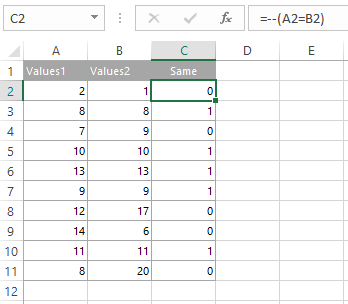# Convert TRUE and FALSE values to 1 and 0 in ExcelHere is a simple trick that is not so obvious. By adding two minus signs before calculation, you can convert TRUE, FALSE into 1, 0 in Excel.

`=--(A2=B2)`